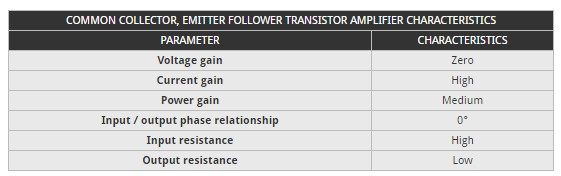# Emitter follower / common collector transistor amplifier Electronics Engineering Notes 1st Year

## Emitter follower / common collector transistor amplifier:

The emitter follower transistor amplifier characteristics enable the circuit to be used as a buffer amplifier.

For both NPN and PNP circuits, it can be seen that for the emitter follower, common collector amplifier circuit, the input is applied to the base, and the output is taken from the emitter. The common terminal for both circuits is the collector.

## Emitter follower transistor amplifier characteristics summary:

The table below gives a summary of the major characteristics of the common collector, emitter follower transistor amplifier.## DC coupled emitter follower, common collector circuit:

The simplest way of connecting an emitter follower is to directly couple the input as shown below. Often the collector of the previous stage will be at approximately the mid rail voltage, and this means that it can be directly coupled to the buffer stage.

The DC coupled emitter follower circuit is particularly easy to design:

1. Choose transistor:   As with other forms of transistor circuit, the transistor should be chosen to meet the anticipated requirements.
2. Emitter resistor value:   The voltage on the emitter is easy to define. It is simply that appearing at the previous stage. Say for example this is half the rail voltage, then the voltage on the emitter Q1 will be 0.5V (for a silicon transistor) less than this – the drop of the base-emitter junction. Simply calculate the value of the resistor for the current required.
3. Emitter follower input resistance:   The input resistance of the circuit is effectively β times the emitter resistor, R1.

## AC coupled emitter follower, common collector circuit:

It is not always possible to directly couple the emitter follower, common collector buffer. When this is the case, it is necessary to add coupling capacitors and bias resistors to the circuit.

The emitter follower can be designed using the design flow below as a basis:

1. Choose transistor:   As before, the transistor type should be chosen according to the anticipated performance requirements.
2. Select emitter resistor:   Choosing an emitter voltage of about half the supply voltage to give the most even range before the onset of any clipping, determine the current required from the impedance of the following stage.
3. Determine base current:   The maximum base current is the collector current divided by β (or hfe which is essentially the same).
4. Determine the base voltage:   The base voltage is simply the emitter voltage plus the base emitter junction voltage – this is 0.6 volts for silicon and 0.2 volts for germanium transistors.
5. Determine base resistor values:   Assume a current flowing through the chain R1 + R2 of around ten times that of the base current required. Then select the correct ratio of the resistors to provide the voltage required at the base.
1. Determine value of input capacitor value:   The value of the input capacitor should equal the resistance of the input circuit at the lowest frequency to give a -3dB fall at this frequency. The total impedance of the circuit will be β times R3 plus any resistance external to the circuit, i.e. the source impedance. The external resistance is often ignored as this is likely to not to affect the circuit unduly.
2. Determine output capacitor value :   Again, the output capacitor is generally chosen to equal the circuit resistance at the lowest frequency of operation. The circuit resistance is the emitter follower output resistance plus the resistance of the load, i.e. the circuit following.
3. Re-evaluate assumptions:   In the light of the way the circuit has developed, re-assess any circuit assumptions to ensure they still hold valid. Aspects such as the transistor choice, current consumption values, etc.
4. Determine value of input capacitor value:   The value of the input capacitor should equal the resistance of the input circuit at the lowest frequency to give a -3dB fall at this frequency. The total impedance of the circuit will be β times R3 plus any resistance external to the circuit, i.e. the source impedance. The external resistance is often ignored as this is likely to not to affect the circuit unduly.
5. Determine output capacitor value :   Again, the output capacitor is generally chosen to equal the circuit resistance at the lowest frequency of operation. The circuit resistance is the emitter follower output resistance plus the resistance of the load, i.e. the circuit following.
6. Re-evaluate assumptions:   In the light of the way the circuit has developed, re-assess any circuit assumptions to ensure they still hold valid. Aspects such as the transistor choice, current consumption values, etc.

The emitter follower circuit is particularly useful for applications where an input high impedance is required. Offering a high input impedance and low output impedance it does not load circuits that may only have a small output capability, or those circuits like oscillators that need a high impedance load to ensure the optimum stability, etc.

## Emitter follower practical aspects

When using the emitter follower circuit, there are a few practical points to note:

• Collector may need decoupling:   On some rare occasions, an emitter follower circuit can oscillate, especially if long leads are present. One of the easiest ways of preventing this is to decouple the collector to ground using connections that are as short as reasonably possible. Values will depend upon the frequency in use. If necessary a small value of resistance can be placed between the collector and the supply rail.
• Input capacitance affects RF:   Although the emitter follower offers a high resistance to any signals, the base-emitter capacitance may reduce the impedance if signals above a few hundred kilohertz are used. This should be remembered when designing the circuit as this can affect any loading levels significantly.

### Feedback is important to us.

error: Content is protected !!